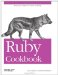# Choosing Randomly from a Weighted List

Problem

You want to pick a random element from a collection, where each element in the collection has a different probability of being chosen.

Solution

Store the elements in a hash, mapped to their relative probabilities. The following code will work with a hash whose keys are mapped to relative integer probabilities:

```	def choose_
weighted(weighted)
sum = weighted.inject(0) do |sum, item_and_weight|
sum += item_and_weight
end
target = rand(sum)
weighted.each do |item, weight|
return item if target <= weight
target -= weight
end
end
```

For instance, if all the keys in the hash map to 1, the keys will be chosen with equal probability. If all the keys map to 1, except for one which maps to 10, that key will be picked 10 times more often than any single other key. This algorithm lets you simulate those probability problems that begin like, "You have a box containing 51 black marbles and 17 white marbles…":

```	marbles = { :black => 51, :white => 17 }
3.times { puts choose_weighted(marbles) }
# black
# white
# black
```

I'll use it to simulate a lottery in which the results have different probabilities of showing up:

```	lottery_probabilities = { "You've wasted your money!" => 1000,
"You've won back the cost of your ticket!" => 50,
"You've won two shiny zorkmids!" => 20,
"You've won five zorkmids!" => 10,
"You've won ten zorkmids!" => 5,
"You've won a hundred zorkmids!" => 1 }

# Let's buy some lottery tickets.
5.times { puts choose_weighted(lottery_probabilities) }
# You've won five zorkmids!
```

Discussion

An extremely naive solution would put the elements in a list and choose one at random. This doesn't solve the problem because it ignores weights altogether: low-weight elements will show up exactly as often as high-weight ones. A less naive solution would be to repeat each element in the list a number of times proportional to its weight. Under this implementation, our simulation of the marble box would contain :black 51 times and :white 17 times, just like a real marble box. This is a common quick-and-dirty solution, but it's hard to maintain, and it uses lots of memory.

The algorithm given above actually works the same way as the less naive solution: the numeric weights stand in for multiple copies of the same object. Instead of picking one of the 68 marbles, we pick a number between 0 and 67 inclusive. Since we know there are 51 black marbles, we simply decide that the numbers from 0 to 50 will represent black marbles.

For the implementation given above to work, all the weights in the hash must be integers. This isn't a big problem the first time you create a hash, but suppose that after the lottery has been running for a while, you decide to add a new jackpot that's 10 times less common than the 100-zorkmid jackpot. You'd like to give this new possibility a weight of 0.1, but that won't work with the choose_ weighted implementation. You'll need to give it a weight of 1, and multiply all the existing weights by 10.

There is an alternative, though: normalize the weights so that they add up to 1. You can then generate a random floating-point number between 0 and 1, and use a similar algorithm to the one above. This approach lets you weight the hash keys using any numeric objects you like, since normalization turns them all into small floating-point numbers anyway.

```	def normalize!(weighted)
sum = weighted.inject(0) do |sum, item_and_weight|
sum += item_and_weight
end
sum = sum.to_f
weighted.each { |item, weight| weighted[item] = weight/sum }
end

lottery_probabilities["You've won five hundred zorkmids!"] = 0.1
normalize!(lottery_probabilities)
# => { "You've wasted your money!" => 0.920725531718995,
# "You've won back the cost of your ticket!" => 0.0460362765859497,
# "You've won two shiny zorkmids!" => 0.0184145106343799,
# "You've won five zorkmids!" => 0.00920725531718995,
# "You've won ten zorkmids!" => 0.00460362765859497,
# "You've won a hundred zorkmids!" => 0.000920725531718995,
# "You've won five hundred zorkmids!" => 9.20725531718995e-05 }
```

Once the weights have been normalized, we know that they sum to one (within the limits of floating-point arithmetic). This simplifies the code that picks an element at random, since we don't have to sum up the weights every time:

```	def choose_
weighted_assuming_unity(
weighted)
target =
rand
weighted.each do |item, weight|
return item if target <= weight
target -= weight
end
end

5.times { puts choose_weighted_assuming_unity(lottery_probabilities) }
# You've won back the cost of your ticket!
```

• Recipe 2.5, "Generating Random Numbers"
• Recipe 6.9, "Picking a Random Line from a File"Ruby Cookbook (Cookbooks (OReilly))
ISBN: 0596523696
EAN: 2147483647
Year: N/A
Pages: 399